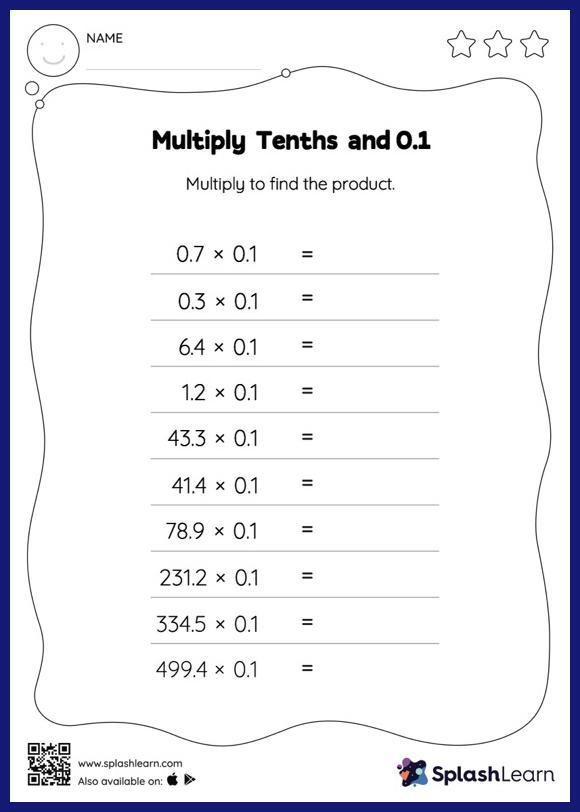# Multiply Tenths and 0.1: Horizontal Multiplication Worksheet

Home > Multiply Tenths and 0.1: Horizontal MultiplicationThis worksheet strengthens students' accuracy and strategic flexibility while they multiply tenths and 0.1. A tenth multiplied by another tenth will result in a product with two decimal digits. As they practice this idea in multiply tenths and 0.1 Worksheet, they first multiply the numbers without accounting for the decimal points and then add the decimal point two places from the right in the product.